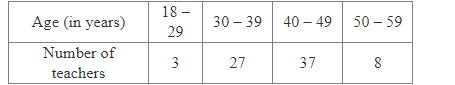# The table given below shows the ages of 75 teachers in a school.

Question:

The table given below shows the ages of 75 teachers in a school.A teacher from this school is chosen at random. What is the probability that the selected teacher is
(i) 40 or more than 40 years old?
(ii) of an age lying between 30 – 39 years (including both)?
(iii) 18 years or more and 49 years or less?
(iv) 18 years or more old?
(v) above 60 years of age?
Note Here 18 – 29 means 18 or more but less than or equal to 29.

Solution:

Total number of teachers = 75

(i) Number of teachers who are 40 or more than 40 years old = 37 + 8 = 45

$\therefore \mathrm{P}($ Selected teacher is 40 or more than 40 years old $)=\frac{\text { Number of teachers who are } 40 \text { or more than } 40 \text { years old }}{\text { Total number of teachers }}=\frac{45}{75}=\frac{3}{5}$

(ii) Number of teachers of an age lying between 30 – 39 years (including both) = 27

∴ P(Selected teacher is of an age lying between 30 – 39 years (including both))

$=\frac{\text { Number of teachers of an age lying between } 30-39 \text { years (including both) }}{\text { Total number of teachers }}=\frac{27}{75}=\frac{9}{25}$

(iii) Number of teachers 18 years or more and 49 years or less = 3 + 27 + 37 = 67

$\therefore P($ Selected teacher is 18 years or more and 49 years or less $)=\frac{\text { Number of teachers } 18 \text { years or more and } 49 \text { years or less }}{\text { Total number of teachers }}=\frac{67}{75}$

(iv) Number of teachers 18 years or more old = 3 + 27 + 37 + 8 = 75

$\therefore \mathrm{P}($ Selected teacher is 18 years or more old $)=\frac{\text { Number of teachers } 18 \text { years or more old }}{\text { Total number of teachers }}=\frac{75}{75}=1$

(v) Number of teachers above 60 years of age = 0

$\therefore \mathrm{P}($ Selected teacher is above 60 years of age $)=\frac{\text { Number of teachers above } 60 \text { years of age }}{\text { Total number of teachers }}=\frac{0}{75}=0$